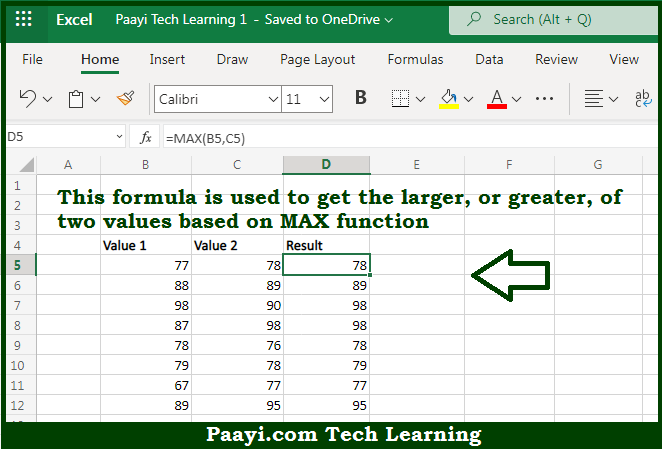# Learn How to Get Large of Two Values in Microsoft Excel

Written by | 0 Comments | 314 Views

In this article, you will learn how to evaluate things with MIN and MAX functions in Microsoft Excel using a single/combination(s) of functions. You will also know to Get Large of Two Values and see the generic formula.

Learn How to Get Large of Two Values in Microsoft Excel

The main purpose of this formula is to get the larger, or greater, of two values. Here we will learn how to get large or two values in the given data range in the workbook in Microsoft Excel. That implies, with the help of a formula based on the MAX function you can able to return the larger, or greater, of two values. So, with the help of this formula, you can able to get a large of two values in the given data range in the workbook in Microsoft Excel.

General Formula to Get Large of Two Values

=MAX(value1,value2)

The Explanation to Get Large of Two ValuesSo we know that with the help of the given formula above you can able to get the larger, or greater, of two values. Here we will learn how to get large or two values in the given data range in the workbook in Microsoft Excel. As we know the MAX function is having complete automatic features. It is used to return the largest value in the numbers range provided. In the example provided here, we give the MAX function two values, and the function will return the larger value amongst the two. So now you have learned how to get large or two values in the given data range in the workbook in Microsoft Excel.